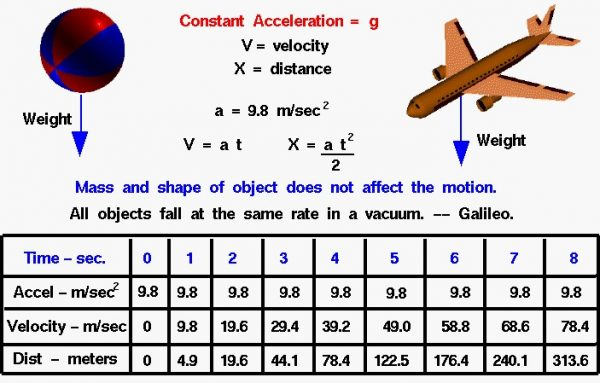# Motion of Free Falling Object## Free Falling

An object that falls through a vacuum is subjected to only one external force, the gravitational force, expressed as the weight of the object. An object that is moving only because of the action of gravity is said to be free falling and its motion is described by Newton’s second law of motion. With algebra we can solve for the acceleration of a free falling object. The acceleration is constant and equal to the gravitational acceleration g which is 9.8 meters per square second at sea level on the Earth. The weight, size, and shape of the object are not a factor in describing a free fall. In a vacuum, a beach ball falls with the same acceleration as an airliner. Knowing the acceleration, we can determine the velocity and location of any free falling object at any time using the following equations.

### X = .5 * a * t^2

where a is the acceleration, V is the velocity, and X is the displacement from an initial location. If the object falls through the atmosphere, there is an additional drag force acting on the object and the physics involved with describing the motion of the object is more complex.

## Table

Here is a table of calculated acceleration (meters per second squared), velocity (meters per second), and displacement (meters) at 1 second intervals.

 Time/Sec 0 1 2 3 4 5 6 7 8 Accel – m/sec2 9.8 9.8 9.8 9.8 9.8 9.8 9.8 9.8 9.8 Velocity – m/sec 0 9.8 19.6 29.4 39.2 49 58.8 68.6 78.4 Distance – meters 0 4.9 19.6 44.1 78.4 122.5 176.4 240.1 313.6

Notice that the acceleration is a constant, the velocity increases linearly, and the location increases quadratically.

## Galileo’s Theory of Motion

The remarkable observation that all free falling objects fall at the same rate was first proposed by Galileo, nearly 400 years ago. Galileo conducted experiments using a ball on an inclined plane to determine the relationship between the time and distance traveled. He found that the distance depended on the square of the time and that the velocity increased as the ball moved down the incline. The relationship was the same regardless of the mass of the ball used in the experiment. The story that Galileo demonstrated his findings by dropping two cannon balls off the Leaning Tower of Pisa is just a legend. However, if the experiment had been attempted, he would have observed that one ball hit before the other! Falling cannon balls are not actually free falling – they are subject to air resistance and would fall at different terminal velocities.

Provide feedback